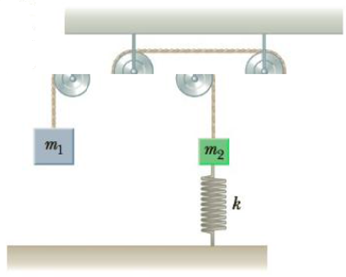Chapter 5, Problem 24P

Chapter
Section
Textbook Problem

Two blocks are connected by a light string that passes over two frictionless pulleys as in Figure P5.24. The block of mass m2 is attached to a spring of force constant k and m1 > m2. If the system is released from rest, and the spring is initially not stretched or compressed, find an expression for the maximum displacement d of m2.Figure P5.24

To determine
The expression for maximum displacement d, of mass m2 .

Explanation

Given Info:

Force constant of spring is k .

The mass m1 is greater than m2 .

In the system, the forces doing work are gravitational force and the spring force. Both are conservative forces.

For a conservative force the total energy will be a constant.

The system is initially at rest and after the maximum upward displacement of the mass m2 , the system is again at rest. Thus, for initial and final states of the system; the kinetic energy is zero.

Since, the total energy of the system is a constant; the gravitational potential energy given by the mass m1 will be equal to the sum of the elastic potential energy stored in the spring when it is stretched a distance d and the gravitational potential energy gained by the mass m2 when it go up a distance d.

Therefore,

m1g|d|=m2g|d|+12k|d<

Still sussing out bartleby?

Check out a sample textbook solution.

See a sample solution

The Solution to Your Study Problems

Bartleby provides explanations to thousands of textbook problems written by our experts, many with advanced degrees!

Get Started

What makes up a system of units?

An Introduction to Physical Science

Why does a stars life expectancy depend on its mass?

Horizons: Exploring the Universe (MindTap Course List)

What impact has recombinant DNA technology had on genetics and society?

Human Heredity: Principles and Issues (MindTap Course List)